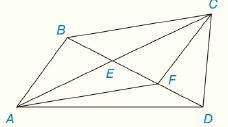Chapter 4.CR, Problem 27CRElementary Geometry For College St...

7th Edition
Alexander + 2 others
ISBN: 9781337614085

Solutions

Chapter
SectionElementary Geometry For College St...

7th Edition
Alexander + 2 others
ISBN: 9781337614085
Textbook Problem

Review Exercises Given: D E is a median of ∆ A D C B E - ≅ F D - E F - ≅ F D - Prove: A B C D is a ▱To determine

To Prove:

The provided quadrilateral ABCF is a parallelogram.

Explanation

Proof:

Definition:

A median of a triangle is a line segment joining a vertex to the midpoint of the opposite side, thus bisecting that side. Every triangle has exactly three medians, one from each vertex, and they all intersect each other at the triangle’s centroid.

Theorem on parallelogram:

If the diagonals of a quadrilateral bisect each other, then it is a parallelogram.

Description:

It is given that DE is a median of ADC with BE-FD- and EF-FD-.

BE-FD-EF-. That is, BE-EF-

Still sussing out bartleby?

Check out a sample textbook solution.

See a sample solution

The Solution to Your Study Problems

Bartleby provides explanations to thousands of textbook problems written by our experts, many with advanced degrees!

Get Started

Evaluate: 182536+47

Elementary Technical Mathematics

Find the limit. limx3x+6xx33x2

Calculus: Early Transcendentals

Solve the equations in Exercises 126. (x+1)2(2x+3)(x+1)(2x+3)2=0

Finite Mathematics and Applied Calculus (MindTap Course List)

Define (a) a polynomial function and (b) a rational function. Give an example of each.

Applied Calculus for the Managerial, Life, and Social Sciences: A Brief Approach

Evaluate the integrals in Problems 7-18. 13.

Mathematical Applications for the Management, Life, and Social Sciences

Prove each identity. sin(90)=cos

Trigonometry (MindTap Course List)

True or False: converges mean exists.

Study Guide for Stewart's Multivariable Calculus, 8th